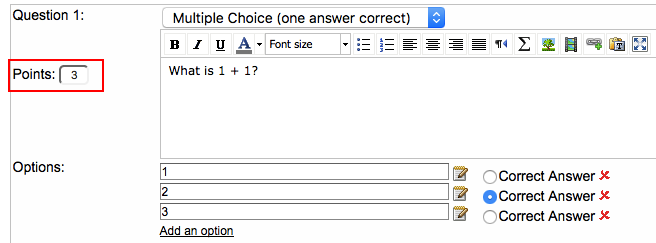Points System

By default the points are calculated like this:
• By default 1 point for every correct gap.
• By default 1 point for every correct multiple choice answer.
• By default 1 point for checked answers (can be changed). A point is only given if all checkboxes are answered correctly, no partial points (can be manually given, see below).
• Essay/open-ended question points are set manually. You can set the number of max points.
How do I change the number of points given for a question?

Change the number of points per question, respectively per gap inside the point box.You can also set half points like this: 0.5.

Manually change calculated points

If you click on the grade icon and then on the student name, you are able to view the responses. Here you can also change the number of given points per answer. If for example you think the student deserves half a point, enter 0.5 in the point box. Then at the bottom of the quiz click the button "Submit Points and Corrections for Essay Questions". This will update the number of total points.More Help